# Simple equation 6

Solve equation with one variable:

X/2+X/3+X/4=X+4

X =  48

### Step-by-step explanation:

X/2+X/3+X/4=X+4

X = 48

Our simple equation calculator calculates it.Did you find an error or inaccuracy? Feel free to write us. Thank you!Tips to related online calculators
Need help to calculate sum, simplify or multiply fractions? Try our fraction calculator.
Do you have a linear equation or system of equations and looking for its solution? Or do you have a quadratic equation?

## Related math problems and questions:

• Equation 25Solve following simple equation: 3/4(x+5)=1/2(x+9)
• Eq2 equationsFor each of the following problems, determine the roots of the equation. Given the roots, sketch the graph and explain how your sketch matches the roots given and the form of the equation: g(x)=36x2-12x+5 h(x)=x2-4x+20 f(x)=4x2-24x+45 p(x)=9x2-36x+40
• EquationsSolve following system of equations: 6(x+7)+4(y-5)=12 2(x+y)-3(-2x+4y)=-44
• Profit, revenue, cost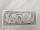Profit, P(x), is the difference between revenue, R(x), and cost, C(x), so P(x) = R(x) - C(x). Which expression represents P(x), if R(x) = 3x3 + 2x - 1 and C(x) = x4 - x2 + 2x + 3?
• Simply equationSolve this equation for x: 9x-6(x-1)=5(x+2)-11
• Expression with powersIf x-1/x=5, find the value of x4+1/x4
• My fatherMy father has a big farm. 6/8 of it were planted with mango trees, 1/2 of the remainder are guava trees and the rest 10 trees are santol trees. What is the number of all trees?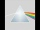Please determine the solvability conditions of the equation, solve the equation and perform the test: x divided by x squared minus 2x plus1 the whole minus x + 3 divided by x squared minus 1 this is equal to 0: x/(x2-2x+1) - (x+3)/( x2-1) = 0
• EqnSolve equation with fractions: 2x/3-50=40+x/4
• If x=4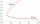If x=4 and y=-3, what is the value of the expression x² + 2xy - 4x + 3y + y²?
• Null points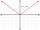Calculate the roots of the equation: 3 |x +4| +3 |x +5| +2 |x +4| = 30
• Equation-3(2-x)-x=2(3x+1)+2x
• Polynomial roots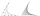Find the other zeroes of the polynomial 2x4+3x3-3x2-6x-2, if two of them are root2 & -root2
• Evaluate 5Evaluate expression x2−7x+12x−4 when x=−1
• Exponential equationSolve for x: (4^x):0,5=2/64.
• Functions f,gFind g(1) if g(x) = 3x - x2 Find f(5) if f(x) = x + 1/2
• Consider 2Consider the following formula: y = 3 ( x + 5 ) ( x - 2 ) Which of the following formulas is equivalent to this one? A. Y=3x2+9x-30 B. Y=x2+3x-10 C. Y=3x2+3x-10 D. Y=3x2+3x-30